Xia, X., Sun, Y., Wu, K., and Jiang, Q. (2014). "Modeling of a straw ring-die briquetting process," BioRes. 9(4), 6316-6328.

#### Abstract

Efficient utilization of biomass resources is crucial for providing renewable energy and mitigating the risk of environmental pollution caused by crop straw burning in China. Straw ring-die briquetting forming is a convenient densification technology to make the low density biomass into briquette fuel; however, the energy consumption of this process is still a challenge. Productivity and torque modeling were carried out in this study on the basis of theoretical derivation. Generally, the straw briquetting process is divided into a compression deformation stage and an extrusion forming stage with the die structure (ring die and roller radius ratio λ) and friction angle φ being the main factors that affect the productivity. Mechanical modeling based on material compaction and calculation modeling based on die-hole pressure were considered for the calculation of σαx in the torque model. A calculation case was then conducted according to the theoretical model, and actual productivity and torque testing were performed for validation purposes. Results show that this deduced productivity model is successful because the deviation is 4.61%. The torque model determined by the calculation model based on die-hole pressure had a better accuracy with a deviation of 6.76%.

Modeling of a Straw Ring-die Briquetting Process

Xianfei Xia, Yu Sun,* Kai Wu, and Qinghai Jiang

Efficient utilization of biomass resources is crucial for providing renewable energy and mitigating the risk of environmental pollution caused by crop straw burning in China. Straw ring-die briquetting forming is a convenient densification technology to make the low density biomass into briquette fuel; however, the energy consumption of this process is still a challenge. Productivity and torque modeling were carried out in this study on the basis of theoretical derivation. Generally, the straw briquetting process is divided into a compression deformation stage and an extrusion forming stage with the die structure (ring die and roller radius ratio λ) and friction angle φ being the main factors that affect the productivity. Mechanical modeling based on material compaction and calculation modeling based on die-hole pressure were considered for the calculation of σαx in the torque model. A calculation case was then conducted according to the theoretical model, and actual productivity and torque testing were performed for validation purposes. Results show that this deduced productivity model is successful because the deviation is 4.61%. The torque model determined by the calculation model based on die-hole pressure had a better accuracy with a deviation of 6.76%.

Keywords: Briquetting; Straw; Productivity; Mechanical modeling; Torque

Contact information: School of Mechanical Engineering, Nanjing University of Science and Technology, No. 200 Xiaolingwei, Nanjing 210094, China;

* Corresponding author: sunyu@njust.edu.cn

INTRODUCTION

With the growing energy crisis and environmental problems (Tuo 2013), utilization of biomass has gained a lot of attention. Because straw contains a large amount of useful lignin and cellulose, efficient utilization of this abundant resource is crucial for providing bio-energy and mitigating the risk of environmental pollution. This research presents a study on the analysis of machinery for handling biomass for energy generation, in particular transforming straw materials to briquettes. The need for this transformation has been widely justified, as shown by other works (Panwar et al. 2010). The transformation has two processes: densifying the low density straw and rolling it to a form for easy transportation. The device to make this transformation is called ring-die briquetting (R-D for short). The R-D system has many advantages such as versatility and flexibility to different types of low density straws compared with the system of screw bar pressing and piston pressing (Stelte et al. 2012), but the shortcoming of high energy consumption during the briquetting process still exists (Zhang et al. 2008).

For all the biomass briquetting systems, the average energy consumption is the most important evaluation index; it is calculated by the ratio of power consumption and productivity (Ståhl and Berghel 2011). Power consumption is the product of speed (ring die rotational speed or roller rotational speed) and torque (Cong et al. 2013), while the speed is generally determined in advance (Yao et al. 2013). When the raw material property remains unchanged, the reasonable parameter design is the main way to reduce energy consumption. So it is necessary to establish the productivity and torque model to describe the energy consumption of the R-D system’s actual working process.

Some studies have focused on the forming mechanism (Adapa et al. 2013; Gillespie et al. 2013), and others on fuel quality (Kaliyan and Morey 2010; Karunanithy et al. 2012). Studies have reported on the energy consumption with this process in a laboratory setting with a single pelleter unit (Ghadernejad and Kianmehr 2012; Hu et al. 2013; Song et al. 2014). It is well known that laboratory studies on such a process can hardly be extended to the industrial setting. Hu (2013) and Holm et al.(2011) obtained the theoretical models to describe the forming pressure by extrusion testing respectively; these models are the bases of force analysis and torque calculation. Duan et al. (2013) made an optimization analysis of energy consumption and productivity of the R-D briquetting machine by factory test, but the corresponding theoretical calculation models were not established. Yao et al. (2013) developed a new vertical R-D briquetting machine to increase productivity and reduce energy consumption; however there was no theoretical calculation of its productivity; and the forming pressure was considered to be constant in the power consumption analysis. Although Ståhl and Berghel (2011) studied the energy consumption of a pilot-scale production of wood fuel pellets, the testing object was a flat die machine.

Cong et al. (2013) and Wu et al. (2013) built up the productivity and torque models of a biomass pellet mill; however the method to calculate the pressure in the forming zone was not comprehensive enough. In addition, there are some obvious differences between a biomass briquetting machine and a pellet mill: the ring die of pellet mill is the driving component and it rotates under normal condition, while the ring die of a briquetting machine is fixed on the frame, and the driving component is roller; the pellet mill ring die is usually horizontal placement, whereas the briquetting machine ring die is vertical. The motion state and force condition of the main working parts are various.

Therefore, the current models are not applicable. Modeling the R-D process to more accurately predict its productivity and driving torque is very important in reasonably designing or optimizing the R-D briquetting machine. The objective of this work was to present a model to calculate the productivity and driving torque for the R-D process for straw material. The paper is organized as follows: first, a brief introduction for the working principle for the straw briquetting process is given. Then, the model for estimating productivity rate and for computing the torque will be presented. Later, a case study with the experiment on an industrial scale is presented to verify the model. Finally, some conclusions are given.

EXPERIMENTAL

The Working Principle of the Straw R-D Process

The working principle of the straw R-D process is shown in Fig. 1. The ring die is fixed on the machine. When the straw material is fed into the cavity formed by the ring die and press roller, the roller (driven by a spindle) starts to rotate and grab the material. The material then is compressed and squeezed into the die holes gradually. Lignin and cellulose contained in the raw material begins to soften and then bond together under the action of frictional heat. After a specific holding time the straw is extruded out as a briquetting fuel with a certain shape (diameter of 20 to 30 mm, length of 40 to 80 mm) and density (0.8 to 1.1 g/cm3).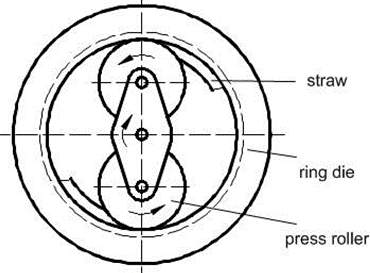Fig. 1. Schematic of the straw R-D process

Model for Estimating the Productivity of the R-D Process

The material grabbing condition

The frictional effect that exists between the ring die, roller, and the material is the key factor that influences the straw briquetting process. Before the material is grabbed, it is in a sliding friction state together with the roller. There are three forces acting on the forming material: the pressure force Nr, the friction force Fr of the roller surface on the straw material, and the pressure force NRof the compressed straw layer on the loose material. The closer the minimum spacing between the roller and ring die, the greater the value of NR. When Fr is greater than the circumferential tangential component of NR, the material is grabbed. The grabbing process is illustrated in Fig. 2a.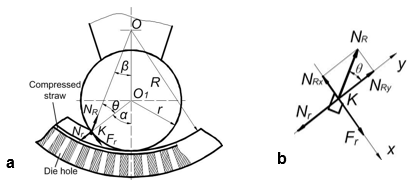Fig. 2. Schematic diagram of the straw grabbing process. (a) The material grabbing process and (b) the force analysis of the grabbed straw

Force analysis is carried out for a small part of material that is about to be grabbed. A coordinate system was established as shown in Fig. 2b. When decomposing NR along the x and ydirections and when the grab condition is met, Eq. 1 is established.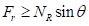(1)

Based on the force equilibrium condition, Nr=NRcosθNr and Frfollow the relationship Fr=fNr, where f is the friction coefficient between the material and the roller, and tanφ=f, where φ is the friction angle. From Eq. 1, Eq. 2 then is set up.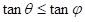(2)

φ is a fixed constant once the material property and roller surface state are determined. In triangle OO1K and according to the sine theorem, β can be determined by Eq. 3.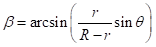(3)

In Fig. 2a, α=β+θ, which means that in the range of α, the straw material is grabbed and compressed. Set R/=λ, and since φ andθ are in the range of 90°, αmax can be expressed as Eq. 4. The value of αmax was affected by friction angle φ and λ and a larger αmax value corresponds to a bigger volume of the compressed raw material. There was a positive correlation between briquetting efficiency and αmax.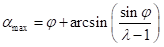(4)

The Model

The variation trend of productivity is considered with reference to the change of α. As shown in Fig. 2, material starts to be grabbed at point K, with a material height of hmax, and hmax=ROK. In triangle OO1KOK can be determined by the cosine theorem, and hmax is calculated by Eq. 5.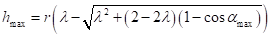(5)

Then productivity Q (average material flow per hour through the ring die) can be calculated by the following Eq. 6,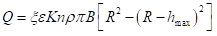(6)

where n is spindle speed (r/min), ρ is material initial density (g/cm3), B is the effective working width of the roller (mm), ε is the opening ratio of the ring die, K is the total number of rollers, ξ is the material effective fill factor, and ξ is the correction factor of Eq. 6 with a general value of 0.4 to 0.7.

Taking Eq. 4 and Eq. 5 into Eq. 6, and unifying the dimensions, the productivity Q can be determined using Eq. 7.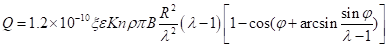(7)

It can be seen from Eq. 7 that the productivity Q is mainly related to die structure (ring die and roller radius ratio λ) and friction angle φ. For the straw R-D machine, the λ value is generally between 2 and 4. The friction coefficient between the straw material and roller are generally between 0.4 and 0.5(φ 20 to 30°). Taking ξ=0.5, ε=0.85, n=160 r/min, B=30 mm, ρ=0.075 g/cm3R=320mm, φ=20~30°, and λ=2~4, and using Eq. 7, the variation of Q is shown in Fig. 3.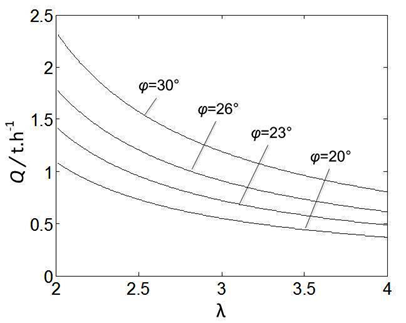Fig. 3. The variation trend of productivity Q

It can be seen from Fig. 3 that a larger φ and smaller λcorresponds to a higher productivity. It should be noted that the actual productivity Q will be different than the theoretical calculation from Eq. 7 due to the impact of material property, uniformity of the feeding process, and other various aspects.

Model for Mechanics of the Briquetting Process

Partition of the forming process

Once the material has been grabbed and completely compressed into the die holes, the forming process can be divided into two phases: the compression deformation stage and the extrusion forming stage. The compression deformation stage can be described as loose material filling the cavity and gradually compacting after the grabbing condition has been met. The pressure of the roller increases rapidly, and then straw material is pushed into a smaller gap region by the frictional force of the roller surface. Material density increases until reaching the requirement during this process. The extrusion forming stage begins once the required density is achieved. Straw is gradually pushed into the die holes under the strong pressure from the roller. The material will not be further compacted in this phase, and the applied pressure is maintained constant and only the shape changes (Wu et al. 2013). The partition is shown in Fig. 4 (0 to α1 is the extrusion forming zone, and α1 to αmax is the compression deformation zone).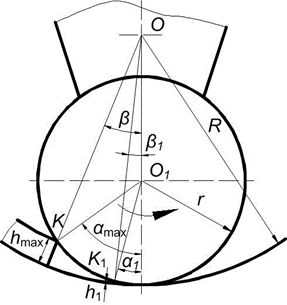Fig. 4. Schematic of the partition

Assuming that the material starts compressing at point K and reaches the required density ρ1 at point K1, the material height here is h1 and wrap angle is α1. Since 2Rh1 and 2R≫h, according to the productivity model, Eq. 8 was established.(8)

Using Eq. (5), h1 can be evaluated; therefore, the value of α1 can be obtained by Eq. (9).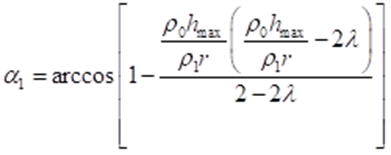(9)

After the partition is determined, a further force analysis of these two areas should be accomplished.

Force analysis of the roller

The active component of the straw R-D machine is the roller, while in the case of a pellet mill it is the ring die. There are some certain differences of the force conditions. The forces acting on the roller include F and N, which are the forces that the driving shaft acts on the center of the roller; N1 and Nrepresent the pressure generated on the surface of the roller and on the two opposite direction friction forces F1 and F2, and are attributed to the material’s compaction in compression deformation and extrusion forming regions respectively. The directions of friction forces F1 and F2 are contrary in the two areas for the following reason: the roller spins around point O driven by the spindle, with the straw material generating the friction force F2 to prevent the roller from turning. Under the action of F2, the roller rotates in the counter-clockwise direction, so the straw material is grabbed and a frictional force F1 opposite to the rotation direction is generated. The force condition is shown as Fig. 5.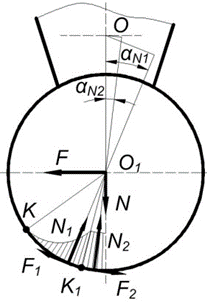Fig. 5Force condition of the roller

According to the moment equilibrium condition in Fig. 5, the moment of each force to point O1 is zero. Then it can be concluded that F1=F2. This means that the frictional force value of the compression deformation and extrusion forming zones are equal. This analysis well explains the rotation behavior of the roller in normal work state.

Solution of driving torque T

In the compression deformation zone, stress σαx increases from zero to the maximum value gradually, but in the extrusion forming zone stress σmax remains constant. In order to determine the value of N1 and N2, force analysis of a small segment of the roller was performed (Jin 2010) and is shown in Fig. 6.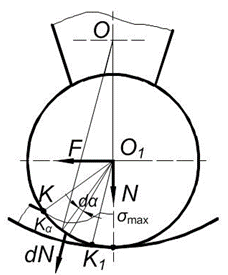Fig. 6. Solving schematic diagram of Nand N2

Assuming that the stress is σαx, then the pressure force dN acting on the roller can be expressed as Eq. 10.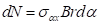(10)

The quantities αN2 and αN1 are the central angles of resultant forces N2 and N1, respectively (Fig. 5). They can be determined by Eqs. 11 and 12.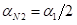(11)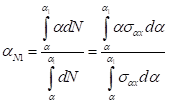(12)

Because the working surface of the roller has a certain curvature, dN should be decomposed along the αN1 (or αN2) direction for solving the resultant force N1 (or N2), as shown in Fig. 7.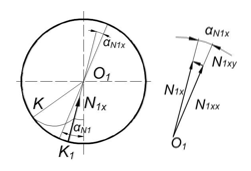Fig. 7. Decomposition diagram of the roller force

N1 and N2 can then be calculated by Eqs. 13 and 14 (Wu and Sun 2013).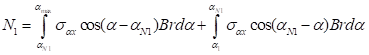(13)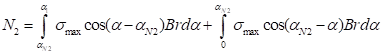(14)

The moment of each force to point O can be represented by Eq. 15.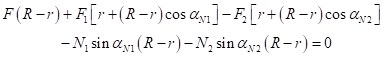(15)

Since F1=F2 and F2=N2fF can be determined by Eq. 16.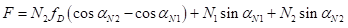(16)

Then, driving torque T can be calculated by Eq. 17.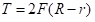(17)

Calculation Model of σαx

Determining the value of torque T requires the solution of σαx. Typically, there are two main calculation methods for determining σαx: a mechanical model based on material compaction and a calculation model based on die-hole pressure. The main differences between these two models are the change trend of σαx and value of σmax.

The mechanical models based on material compaction, model 1, are listed in Table 1. An exponential relationship has been discovered between pressure and compacted density, as Table 1 shows.

Table 1. Mechanical Model Based on Material Compaction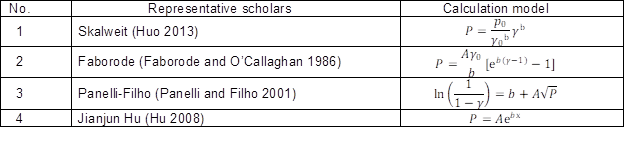Note: P is pressure, γ is compacted density, p0 is initial stress, γ0is initial density, A and b are test coefficients, and x is compaction displacement

In the Hu model (Hu 2008), rice straw was compressed and the test coefficients

were obtained. The research object in this study was rice straw, so this model was adopted and σαx can be described by Eq. 18.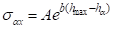(18)

Based on the elastic-plastic mechanic theory, Holm et al. (2011) provides an effective method for calculating the die-hole pressure (Model 2). The pressure σmax at the inlet of the die hole can therefore be calculated by Eq. (19).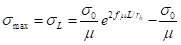(19)

where μ is Poisson’s ratio; σ0 is pre-stressing pressure; f is friction coefficient; rh is die hole diameter; and L is die hole length. The assumption: raw material is elastic and orthotropic material; the differential force is constant over the cross-sectional area πr2.

Wu et al. (2013) indicated that the change law of pressure σαx is in accordance with a quadratic curve model within the scope of α1 to α. The assumptions were as follows: ring die and roller were rigid bodies; body force was not taken into account; the material was distributed on the ring die uniformly; and the modified Drucker-Prager-Cap model was adapted. So σαx can be calculated by Eq. 20 (ht=hmax – h1).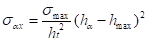(20)

A case analysis of the theoretical model

According to the formulas above, a theoretical calculation was carried out in accordance with the actual parameters. Calculation conditions are as follows. Equipment structure parameters: = 320 mm, = 720 mm, = 30 mm, = 165 mm, and r= 30 mm. Material property parameters: ρ0 = 0.075 g/cm3ρ= 1.02 g/cm3φ = 25° (= 0.466), and = 0.45. Parameters of the Hu model are = 0.1002 and = 0.0710 (Hu 2008). The correction factor ζ in Eq. (6) and pre-stressing pressure σin Eq. (18) have been obtained through testing prior to the calculation (ζ is 0.47 and σ0 is 0.1895 MPa). Variation of σαx based on two models in the compression deformation zone is shown as Fig. 8. Calculation results of the two models are shown in Table 2.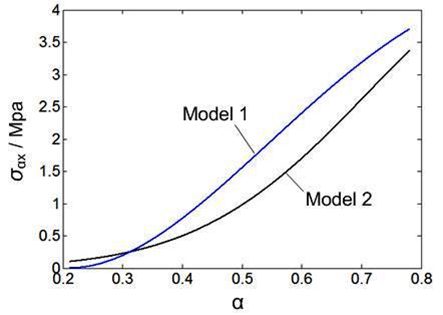Fig. 8. Variation of σαx in the compression deformation

Table 2. Calculation Results of the Two Models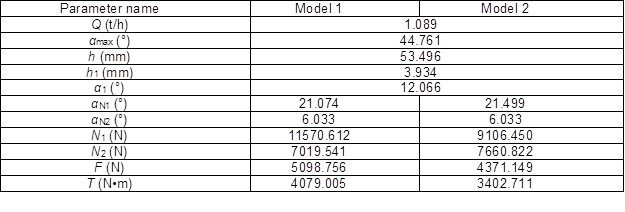Experimental Verification

To verify the accuracy of the theoretical model, productivity and torque testing were completed. Productivity testing was performed by digital electronic weight (according to the agricultural industry criteria of China NY/T 1883-2010), and torque testing of the drive motor’s shaft was implemented by the Bichuang wireless torque measurement system (Beijing Bichuang Technology Co., Ltd., Beijing, China). In this system, strain gauges were symmetrically arranged to eliminate the impact of bending moment. The arrangement method and bridging form of strain gauge are shown in Fig. 9a.

Experimental equipment and materials include a ring-die straw briquetting machine (9EYK1800; Jiangsu ENERGY Renewable Resources Co., Ltd., Taizhou, Jiangsu Province, China), wireless nodes (TQ201; Beijing Bichuang Technology Co., Ltd., Beijing, China), digital electronic weighter, special torque strain gages, wireless gateway, special torque analysis software, sandpaper, alcohol cotton, glue, scissors, and fiber tape. The raw material was rice straw harvested in the autumn of 2013 in Jiangyan, Jiangsu Province, China. The straw was chopped, with particle size of 2 to 20 mm and moisture content of 15 to 21%, and briquetted without adding any binders. Experiments were conducted at an industrial factory (Jiangsu ENERGY Renewable Resources Co., Ltd., Taizhou, Jiangsu Province, China). Figure 9b shows the torque testing site.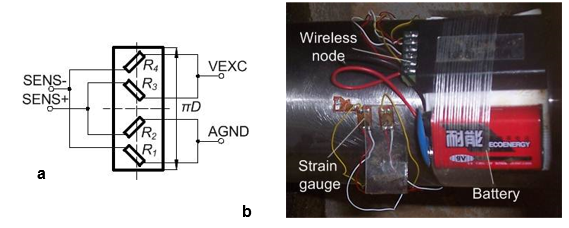Fig. 9. Torque testing program. (a) Arrangement and bridging form of strain gauge and (b) torque testing site

RESULTS AND DISCUSSION

Figure 10 shows the torque testing data. The average value of the motor shaft’s testing torque TM was 0.729 kN•m and the roller spindle’s testing torque T was 3.187 kN•m (= iηTM, where η is transmission efficiency and i is transmission ratio; = 4.6, η = 0.95 for the testing machine).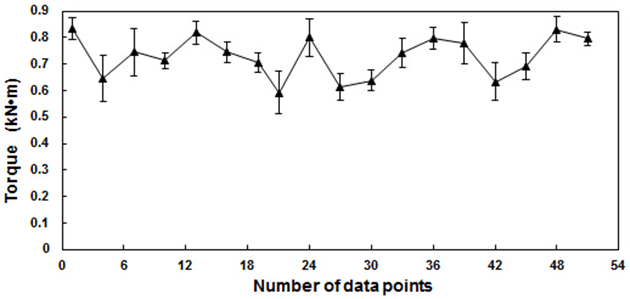Fig. 10. Experimental torque data. Data is expressed as average ± SD

Experimental results and deviations are shown in Table 3. The testing value of productivity Q was 1.041 t/h, the average of three test results. There was a deviation (between the calculated and tested values) of 4.61% due to the impact of the feeding process (rice straw contains lignin and cellulose; they are very soft and can easily entwine together. Therefore, small groups of straw material are formed one after another during the feeding). Fluctuation of torque in Fig. 10 shows the non-uniformity of the actual feeding process. Therefore, the deviation is within an acceptable range. Compared with Model 1, Model 2 exhibited a better accuracy with the deviation (between the calculated and tested values) of 6.76%, which means that Model 2 can describe the mechanical behavior of straw actual compression process more accurately.

Table 3. Experimental Results and Deviation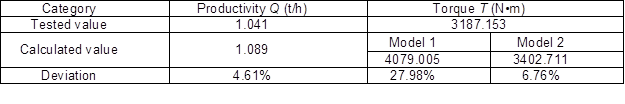CONCLUSIONS

This work was aimed at reducing the energy consumption and improving the efficiency of the straw briquetting process. Based on the theoretical derivation and experimental validation, the key conclusions are summarized as follows:

1. Productivity Q is higher at smaller die structure (radius ratio λ) and larger friction angle φ. The forming process can be divided into two stages: compression deformation and then extrusion forming. Two stages are corresponding at 0 to α1 and αto αmax during the process, respectively.
2. The forces acting on the roller include and N, for which the driving shaft acts on the center of the roller; N1 and N2represent the pressure generated on the surface of the roller and on the two opposite direction friction forces F1 and F2.
3. In the compression deformation zone, stress σαincreases from zero to the maximum value gradually, but in the extrusion forming zone stress σmax remains constant. There are two main calculation methods for σαx: a mechanical model based on material compaction and a calculation model based on die-hole pressure.
4. Experimental validation indicates that the productivity model was successful since the deviation was calculated to be 4.61%. The torque model determined by the calculation model based on the die-hole pressure had a better accuracy with a deviation of 6.76%.

ACKNOWLEDGMENTS

This work was supported by The Project of Six Talents Peak of Jiangsu Province (2010-JXQC-080), The Natural Science Foundation of Jiangsu Province (BK2011706), and The Prospective Industry-Study-Research Cooperation Foundation of Jiangsu Province (BY2012023).

REFERENCES CITED

Adapa, P. K., Tabil, L. G., and Schoenau, G. J. (2013). “Factors affecting the quality of biomass pellet for biofuel and energy analysis of pelleting process,” Int. J. Agric. Biol. Eng. 6(2), 1-12.DOI: 10.3965/j.ijabe.20130602.001

Cong, H. B., Zhao, L. X., Yao, Z. L., Tian, Y. S., and Meng, H. B. (2013). “Analysis on capacity and energy consumption of biomass circular mould granulator,” Trans. Chin. Soc. Agric. Machinery (Nongye Jixie Xuebao) 44(11), 144-149.

Duan, J., Chen, S. R., Yao, Y., and Jiang, X. X. (2013). “Energy consumption test and process optimization for circular mold briquetting machine,” Trans. Chin. Soc. Agric. Machinery (Nongye Jixie Xuebao) 44(S1), 149-155.

Faborode, M. O., and O’Callaghan, J. R. (1986). “Theoretical analysis of the compression of fibrous agricultural materials,” J. Agric. Eng. Res. 35(3), 175-191. DOI: 10.1016/S0021-8634(86)80055-5

Ghadernejad, K., and Kianmehr, M. H. (2012). “Effect of moisture content and particle size on energy consumption for dairy cattle manure pellets,” Agric. Eng. Int.: CIGR J. 14(3), 125-130.

Gillespie, G. D., Everard, C. D., Fagan, C. C., and McDonnell, K. P. (2013). “Prediction of quality parameters of biomass pellets from proximate and ultimate analysis,” Fuel 111, 771-777. DOI: 10.1016/j.fuel.2013.05.002

Holm, J. K., Stelte, W., Posselt, D., Ahrenfeldt, J., and Henriksen, U. B. (2011). “Optimization of a multiparameter model for biomass pelletization to investigate temperature dependence and to facilitate fast testing of pelletization behavior,” Energ. Fuel 25(8), 3706-3711. DOI: 10.1021/ef2005628

Huo, L. L. (2013). Study on Forming Mechanism of Biomass Pellet and Process Optimization of Pellet Mill, Ph.D dissertation, China Agricultural University, Beijing, China.

Hu, J. J. (2008). “Straw pellet fuel cold molding by compression: Experimental study and numerical simulation,” Ph.D dissertation, Dalian University of Technology, Dalian, China.

Hu, J. J., Lei, T. Z., Sheng, S. Q., and Zhang, Q. G. (2013). “Specific energy consumption regression and process parameters optimization in wet-briquetting of rice straw at normal temperature,” BioResources 8(1), 663-675.

Jin, Z. (2010). Engineering Mechanics, Southeast University Press, Nanjing, China.

Karunanithy, C., Wang, Y., Muthukumarappan, K., and Pugalendhi, S. (2012). “Physicochemical characterization of briquettes made from different feedstocks,” Biotechnol. Res. Int. 2012, 1-12. DOI:10.1155/2012/165202

Kaliyan, N., and Morey, R. V. (2010). “Densification characteristics of corn cobs,” Fuel Process Technol. 91(5), 559-565. DOI: 10.1016/j.fuproc.2010.01.001

Panelli, R., and Filho, F. A. (2001). “A study of a new phenomenological compacting equation,” Powder Technol. 114(1), 255-261. DOI: 10.1016/S0032-5910(00)00207-2

Panwar, V., Prasad, B., and Wasewar, K. L. (2010). “Biomass residue briquetting and characterization,” J. Energ. Eng.-ASCE137(2), 108-114. DOI: 10.1061/(ASCE)EY.1943-7897.0000040

Song, X., Zhang, M., Pei, Z. J., and Wang, D. (2014). “Ultrasonic vibration-assisted pelleting of wheat straw: A predictive model for energy consumption using response surface methodology,” Ultrasonics 54(1), 305-311. DOI: 10.1016/j.ultras.2013.06.013

Ståhl, M. and Berghel, J. (2011). “Energy efficient pilot-scale production of wood fuel pellets made from a raw material mix including sawdust and rapeseed cake,” Biomass & Bioenerg.35(12), 4849-4854. DOI: 10.1016/j.biombioe.2011.10.003

Stelte, W., Sanadi, A. R., Shang, L., Holm, J. K., Ahrenfeldt, J., and Henriksen, U. B. (2012). “Recent developments in biomass pelletization – A review,” BioResources 7(3), 4451-4490.

Tuo, H. F. (2013). “Energy and energy-based working fluid selection for organic Rankine cycle recovering waste heat from high temperature solid oxide fuel cell and gas turbine hybrid systems,” Int. J. Energ. Res. 37(14), 1831-1841. DOI: 10.1002/er.3001

Wu, K., Sun, Y., Peng, B. B., Ding, W. X., and Wang, S. H. (2013). “Modeling and experiment on rotary extrusion torque in ring-die pelleting process,” Trans. Chin. Soc. Agric. Eng. (Nongye Gongcheng Xuebao) 29(24), 33-39. DOI: 10.3969/j.issn.1002-6819.2013.24.005

Wu, K., and Sun, Y. (2013), Ring-die Pellet Forming Technology and Equipment, Science Press, Beijing, China.

Yao, Z. L., Zhao, L. X., Tian, Y. S., Meng, H. B., and Pang, L. S. (2013). “Study on biomass briquetting machines with vertical ring die,” Trans. Chin. Soc. Agric. Machinery (Nongye Jixie Xuebao) 44(11), 139-143. DOI: 10.6041/j.issn.1000-1298.2013.11.025

Zhang, B. L., Wang, X. T., and Yang, S. G. (2008). “Key problems in production and application of straw densification briquetting fuel (SDBF),” Trans. Chin. Soc. Agric. Eng. (Nongye Gongcheng Xuebao) 24(7), 296-300.

Article submitted: July 10, 2014; Peer review completed: August 9, 2014; Revised version received: August 25, 2014; Accepted: August 26, 2014; Published: September 2, 2014.